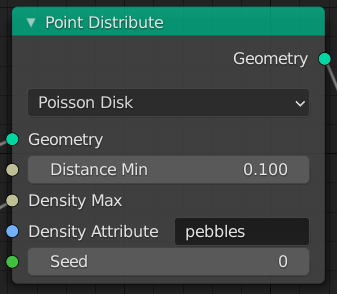# Point Distribute¶The Point Distribute Node.

The Point Distribute node places points on the surface of the input geometry object. Point, corner and polygon attributes of the input geometry are transferred to the generated points. That includes vertex weights and UV maps. Additionally, the generated points will have a normal, id and rotation attribute.

## Inputs¶

Geometry

Standard geometry input.

Note

The input geometry must contain a mesh with faces.

Distance Min

The minimal distance points can have to each other. This option is only available on distribution methods that support it.

Density Max

The point density for the point distribution. The units are number of points per square meter. This value is multiplied by the values from the Density Attribute.

Note

This will be capped on distributions by the Distance Min option. If the density is greater than what the minimal distance allows, no new points will be added after this threshold has been passed.

Density Attribute

The name of the attribute to use for influencing the point density. The values of this attribute are multiplied by Density Max for the final density value.

Seed

The random Seed to use when generating points.

## Properties¶

Distribution Method
Random

Distribute points randomly on the surface. This is the fastest distribution method.

Poisson Disk

Distribute points randomly on the surface while taking a minimum distance into account.

## Output¶

Geometry

Generated points. Several attributes are created on the output points based on the input mesh:

`id (int)`

An identifier for each point used for stability when the mesh is deformed, used in the Attribute Randomize and Point Instance nodes.

`normal (vector)`

The Normal of the triangle on which each point is scattered.

`rotation (vector)`

An XYZ Euler rotation built from the normal attribute for convenience.